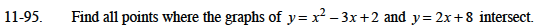### Home > CAAC > Chapter 11 > Lesson 11.2.2 > Problem11-95

11-95.Use the Equal Values Method and solve for x.

x2 − 3x + 2 = 2x + 8
x2 − 5x + 2 = 8
x2 − 5x − 6 = 0

Factor and use the Zero Product Property to find the values for x.

Substitute the values for x back into one of the original equations to solve for y.

The points of intersection are (6, 20) and (−1, 6).